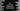# C++ iswlower() method explanation with example## C++ iswlower() method explanation with example:

iswlower method is defined in the cwtype.h header file. iswlower method can be used to check if a character is lowercase character or not.

We can use this method to quickly find out if a character is lowercase character or not.

### iswlower method definition:

iswlower method is defined as like below:

``int iswlower(wint_t c)``

This method checks if a character is lowercase or not.

### Parameters of iswlower:

This method takes one character c as the parameter and checks if that character is lowercase character or not.

### Return value of iswlower:

This method returns a non-zero value if c is a lowercase character. It returns 0 if c is not a lowercase character.

## Example of iswlower:

Let’s take an example of iswlower and try it with different

``````#include <cwctype>
#include <iostream>
using namespace std;

int main()
{
wchar_t arr[] = {'a', 'B', 'c', 'Z', 'X', '@', '*'};

for (int i = 0; i < 7; i++)
{
cout << char(arr[i]) << " : " << iswlower(arr[i]) << endl;
}
}``````

Here,

• We are importing cwctype header file as iswlower is defined in this header file.
• arr is an array of characters. This includes both lower-case and upper-case characters.
• The for loop runs from index 0 to 7, i.e. it iterates over the characters of the array.
• Inside the loop, we are printing the currently iterating character and the result of iswlower.

If you run this program, it will print:

``````a : 1
B : 0
c : 1
Z : 0
X : 0
@ : 0
* : 0``````

As you can see here, it is printing 1 only for lower case characters.

You can also use it with any conditional statement since 1 and 0 are considered as true and false.

For example,

``````#include <cwctype>
#include <iostream>
using namespace std;

int main()
{
wchar_t arr[] = {'a', 'B', 'c', 'Z', 'X', '@', '*'};

for (int i = 0; i < 7; i++)
{
if (iswlower(arr[i]))
{
cout << char(arr[i]) << " is a lowercase character" << endl;
}
else
{
cout << char(arr[i]) << " is a uppercase character" << endl;
}
}
}``````

Inside the loop, we have added one if-else block in this example. If iswlower return 1, it will move inside the if block and if it return 0, it will move inside the else block.

If you run this program, it will print:

``````a is a lowercase character
B is a uppercase character
c is a lowercase character
Z is a uppercase character
X is a uppercase character
@ is a uppercase character
* is a uppercase character``````